### Adding a Change size node

This node changes the size and resolution of a terrain.

To add a Change size node, right click in the Graph Editor and select Create Node  > Terrain Transformation  > Change size.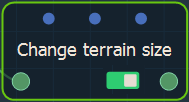Double click on the node to open its parameters: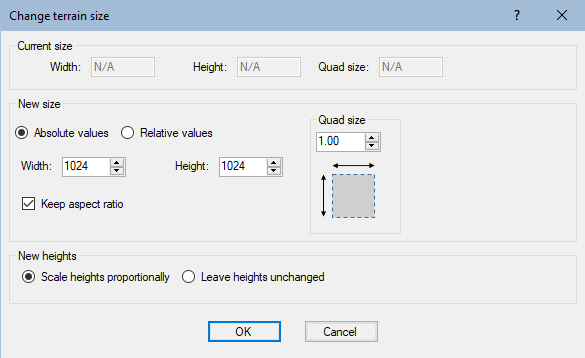### Changing the Terrain size

The Current size in the parameters dialog displays the current Terrain Width and Height in meters and the Quad size of a grid quad.

• Use the New size fields to change the  Width and Height in meters and the Quad size of the Terrain.
• Toggle between Absolute values (meters) and Relative values (%).
• Check the Keep aspect ratio box to preserve the proportions of the Terrain when you change one value.
• Scale heights proportionally: The scale factor applied to the width and the height is also applied to the heights of the vertices so that the terrain keeps with the same look. This means for example that the slopes angle is not altered.
The scale factor is applied to the heights of the vertices following these rules:
• If both dimensions are increased, the smallest one is applied to the scaling factors.
• If both dimensions are decreased, the largest one is applied to the scaling factors.
• Otherwise, the heights cannot be changed (scale factor = 1).
• Leave heights unchanged: The heights of the vertices are preserved.

### Linking to an input node

Link the Smooth node to an input node.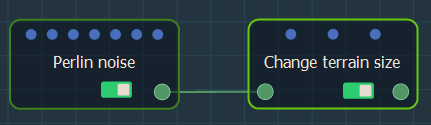The size of the terrain is divided by 2 and the Scale heights accordingly parameter is selected. The output terrain is a smaller version of the original terrain.

For example: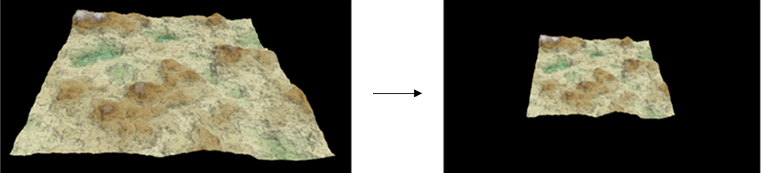In this example, the size of the terrain is divided by 2 and the Leave heights unchanged parameter is selected. The output terrain is smaller but the mountains have still the same heights; now they are sharper.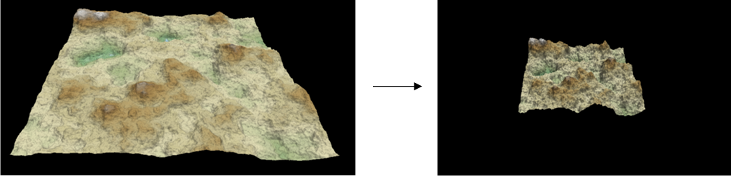### Parameters

Parameter Use
Width New width (in meters) or the scale factor (as a percentage) to be applied to the width
Height New height (in meters) or the scale factor (as a percentage) to be applied to the height
Quad size New size (in meters) of a grid quad
Absolute values Defines the values of the new dimensions of the Terrain in meters
Relative values Sets the scale factor to be applied to the dimensions of the Terrain as a percentage
Keep aspect ratio If selected, the width to height ratio is constrained
Scale heights proportionally The scale factor applied to the width and the height is also applied to the heights of the vertices so that the terrain remains with the same look.
Leave heights unchanged The heights of the vertices are preserved